×

Search anything:

# Instance Segmentation

#### Deep Learning Machine Learning (ML)In this article, we will dive deeper in a very important concept in computer vision which considered a great progress in deep learning field which is Instance Segmentation. what is it?, the most popular algorithm for it, and its evaluation metric.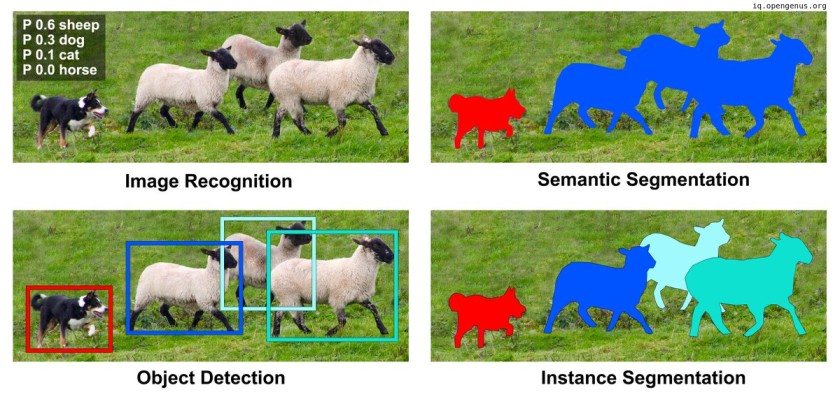#### 4. Evaluation metrics for it.

Hoping this illustration will be enough for most of you to understand the fundamentals of Instance Segmentation as it is a very common and critical concept in the machine learning field as in the computer vision sector.

#### 1. What is Instance Segmentation :

First of all we want to know "what is the instance segmentation" ? well, we already know what is object detection and that it try to detect a variable numbers of objects and there locations in each image by adding bounding box around each object. From this point instance segmentation will start by combining a semantic segmentation approach to the object detection one, So instead of just a bounding box a round the object, now will be a precise mask a round each object and also differentiate between instances of the same class. so we can define the Instance Segmentation as : A combination of object detection that detect more than one object in the image and semantic segmentation that put a precise mask on each object leading to differentiation of each instance in the same class.!

2022_12_23_0lg_Kleki

In the above image the bounding boxes come from object detection and the colored masks come from semantic segmentation.

#### 2. Instance Segmentation methods

There are two famous approaches for obtaining instance segmentation :

1. proposal-based method.
2. FCN-based method.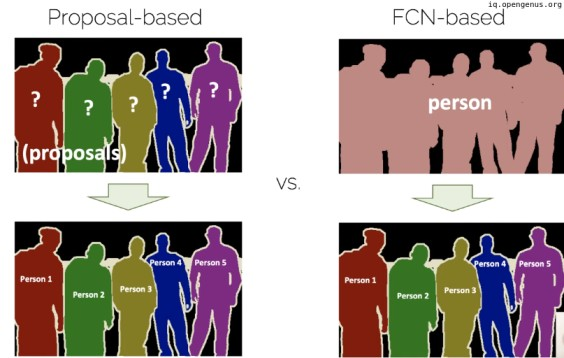In the first method, we will start by take the knowledge we got from object detection So, we will start with object proposals and then assign a class to each instance to it.(we will talk about it latter).

while in the second approach we start from semantic segmentation where each pixel is assigned to a class then applying another approaches to separate each instance may be through clustering each instance pixels."called bottom-up or proposal-free approach.

So far the proposal based method showed greater performance the the proposal free one so in this section we will talk about the most famous algorithm for it which is "Masked Region based Convolution Neural Networks" or Mask R-CNN for short :
As we said earlier that instance segmentation consist of two phases "object detection and semantic segmentation" so the algorithm will consist of two parts :

1. Faster R-CNN for object detection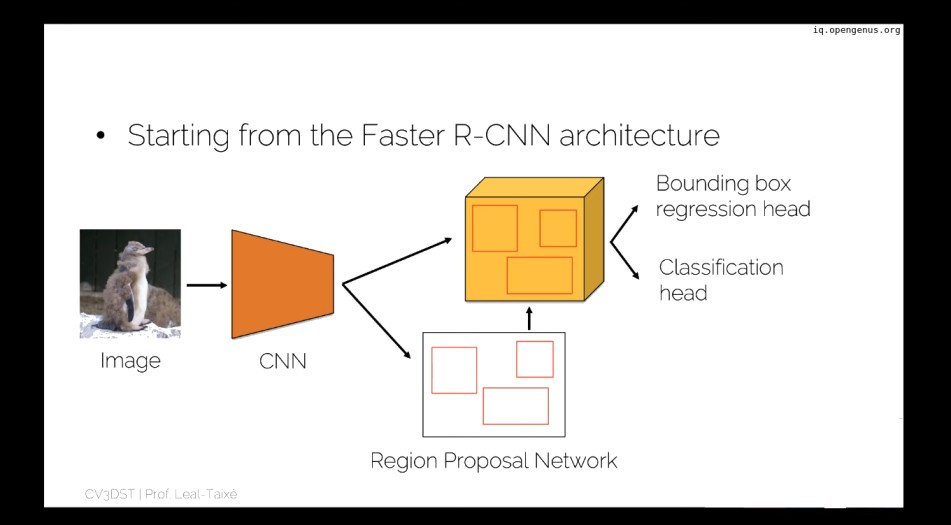1. FCN for semantic segmentation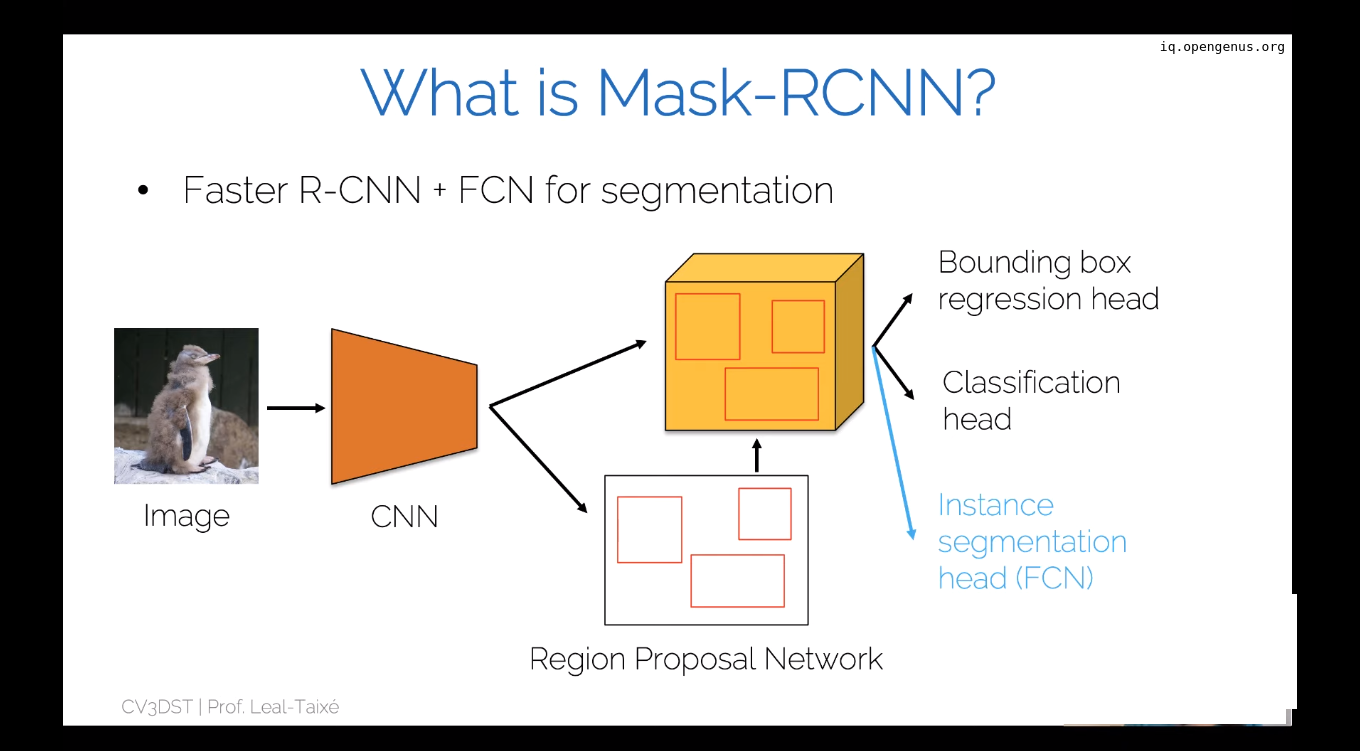it is obviously that the image enter CNN layer producing a feature map that enter a regional proposal network(RPN) for ROI predictions the ROI align process occurred the entering the three parallel heads of bounding box, classification (Dense layer) and instance segmentation (FCN).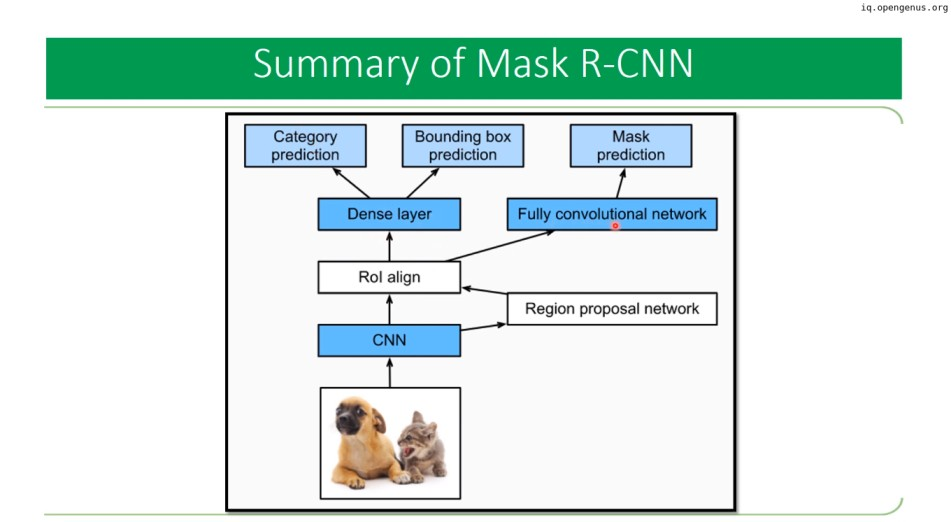#### 4. Evaluation metrics for it :

There are many of evaluation metrics for instance segmentation like IoU (intersection over union), recap (TP/Ground Truth), precision (TP/ Positive Predictions) but the most important metric is the mean average precision (mAP) :
which is the area under the curve of recall and precision curve after arrangement the predictions according to confidence level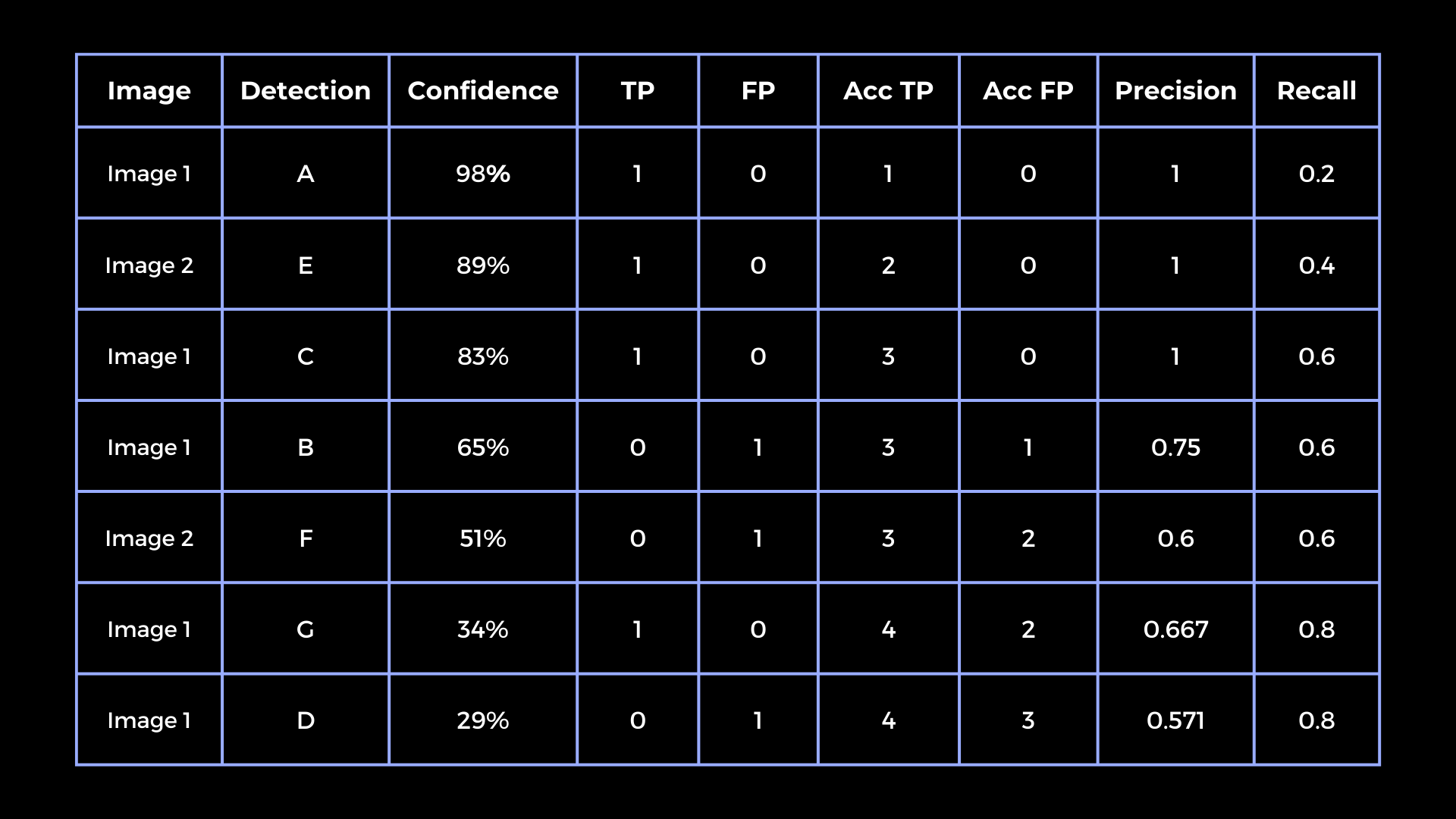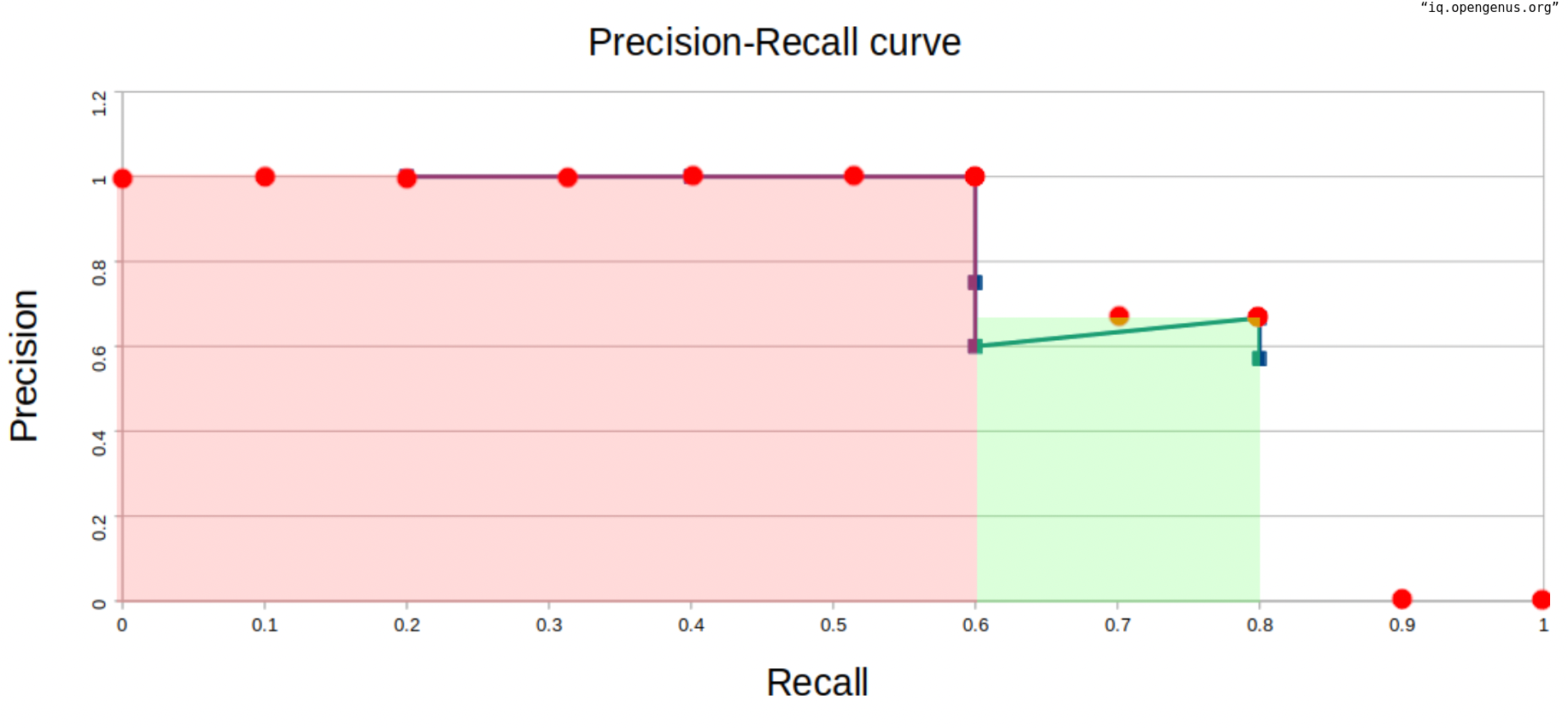then get the mean for all classes .

Also you can use tensorflow and keras to provide it to you :

``````tfr.keras.metrics.MeanAveragePrecisionMetric(
name=None, topn=None, dtype=None, ragged=False, **kwargs)

``````

With this article at OpenGenus, you must have the complete idea of Instance Segmentation.